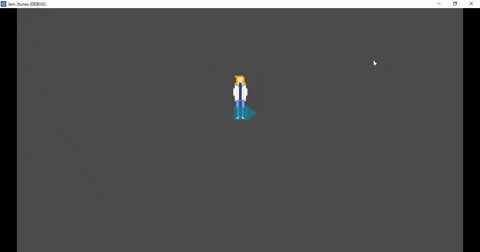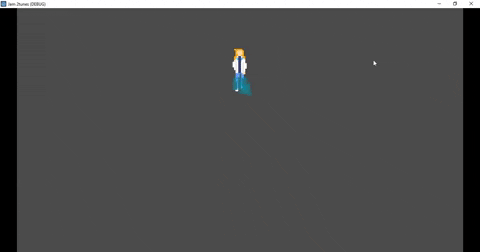How can I make the raycast's rotation_degrees be equal the player's direction?
Like when the player goes in diagonal direction and then the raycast points in the same direction.Hi,
Make the Raycast a child of the sprite

or

something like:
`\$RayCast2D.global_rotation = \$Sprite.global_rotation`

answered Jul 21 by (1,261 points)

I tried both methods and no one works :/Its like this on the tree and nothing happens if I put the raycast as a children of the sprite.

Here's the script of the player:

``````extends KinematicBody2D

onready var anim : AnimationPlayer = \$Sprite/anims
var frame = 0
export (int) var speed = 70
var pode_andar = true
var movendo
var mover : Vector2

func input():
mover.x = Input.get_action_strength("direita") - Input.get_action_strength("esquerda")
mover.y = Input.get_action_strength("baixo") - Input.get_action_strength("cima")

if abs(mover.x) == 1 and abs(mover.y) == 1:
mover = mover.normalized()
movendo = speed * mover

# sprites da movimentação
if mover.x == 1:
anim.play("direita")
frame = 4
if mover.x == -1:
anim.play("esquerda")
frame = 10
if mover.y > 0:
anim.play("baixo")
frame = 7
if mover.y < 0:
anim.play("cima")
frame = 1
if mover.x == 0 and mover.y == 0:
\$Sprite/anims.stop()
\$Sprite.frame = frame
move_and_slide(movendo)

func _physics_process(delta):
if pode_andar:
input()
raycast()

func raycast():
\$RayCast2D.global_rotation = mover.angle()
``````

With the "func raycast", the arrow points where I want, but when I stop move, the arrow returns to the initial point.

Hi,
I had to subtract 90 degrees to get it to point the correct way in my test.
Is this what you wanted?
You'll have to log the last direction and set that to the correct angle when the player doesn't move.

`````` func raycast():
``````When I walk, the arrow points correctly, but when I stop, still the same: the arrow points to the initial point

Yes, I did mention that.

A quick fix:

Set `mover` to zero before changing the values:

``````func input():
mover = Vector2.ZERO
``````

Only change the raycast angle if you've moved:

``````func raycast():
if mover != Vector2.ZERO: